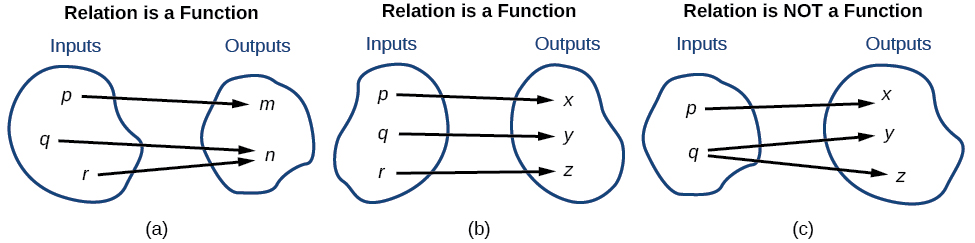# 3.1 Functions and function notation

 Page 1 / 21
In this section, you will:
• Determine whether a relation represents a function.
• Find the value of a function.
• Determine whether a function is one-to-one.
• Use the vertical line test to identify functions.
• Graph the functions listed in the library of functions.

A jetliner changes altitude as its distance from the starting point of a flight increases. The weight of a growing child increases with time. In each case, one quantity depends on another. There is a relationship between the two quantities that we can describe, analyze, and use to make predictions. In this section, we will analyze such relationships.

## Determining whether a relation represents a function

A relation    is a set of ordered pairs. The set consisting of the first components of each ordered pair    is called the domain and the set consisting of the second components of each ordered pair is called the range . Consider the following set of ordered pairs. The first numbers in each pair are the first five natural numbers. The second number in each pair is twice that of the first.

$\left\{\left(1,\text{\hspace{0.17em}}2\right),\text{\hspace{0.17em}}\left(2,\text{\hspace{0.17em}}4\right),\text{\hspace{0.17em}}\left(3,\text{\hspace{0.17em}}6\right),\text{\hspace{0.17em}}\left(4,\text{\hspace{0.17em}}8\right),\text{\hspace{0.17em}}\left(5,\text{\hspace{0.17em}}10\right)\right\}$

The domain is $\left\{1,\text{\hspace{0.17em}}2,\text{\hspace{0.17em}}3,\text{\hspace{0.17em}}4,\text{\hspace{0.17em}}5\right\}.$ The range is $\left\{2,\text{\hspace{0.17em}}4,\text{\hspace{0.17em}}6,\text{\hspace{0.17em}}8,\text{\hspace{0.17em}}10\right\}.$

Note that each value in the domain is also known as an input value, or independent variable    , and is often labeled with the lowercase letter $\text{\hspace{0.17em}}x.\text{\hspace{0.17em}}$ Each value in the range is also known as an output value, or dependent variable    , and is often labeled lowercase letter $\text{\hspace{0.17em}}y.$

A function $\text{\hspace{0.17em}}f\text{\hspace{0.17em}}$ is a relation that assigns a single element in the range to each element in the domain . In other words, no x -values are repeated. For our example that relates the first five natural numbers    to numbers double their values, this relation is a function because each element in the domain, $\left\{1,\text{\hspace{0.17em}}2,\text{\hspace{0.17em}}3,\text{\hspace{0.17em}}4,\text{\hspace{0.17em}}5\right\},$ is paired with exactly one element in the range, $\left\{2,\text{\hspace{0.17em}}4,\text{\hspace{0.17em}}6,\text{\hspace{0.17em}}8,\text{\hspace{0.17em}}10\right\}.$

Now let’s consider the set of ordered pairs that relates the terms “even” and “odd” to the first five natural numbers. It would appear as

$\left\{\left(\text{odd},\text{\hspace{0.17em}}1\right),\text{\hspace{0.17em}}\left(\text{even},\text{\hspace{0.17em}}2\right),\text{\hspace{0.17em}}\left(\text{odd},\text{\hspace{0.17em}}3\right),\text{\hspace{0.17em}}\left(\text{even},\text{\hspace{0.17em}}4\right),\text{\hspace{0.17em}}\left(\text{odd},\text{\hspace{0.17em}}5\right)\right\}$

Notice that each element in the domain, $\left\{\text{even,}\text{\hspace{0.17em}}\text{odd}\right\}$ is not paired with exactly one element in the range, $\left\{1,\text{\hspace{0.17em}}2,\text{\hspace{0.17em}}3,\text{\hspace{0.17em}}4,\text{\hspace{0.17em}}5\right\}.$ For example, the term “odd” corresponds to three values from the domain, $\left\{1,\text{\hspace{0.17em}}3,\text{\hspace{0.17em}}5\right\}$ and the term “even” corresponds to two values from the range, $\left\{2,\text{\hspace{0.17em}}4\right\}.$ This violates the definition of a function, so this relation is not a function.

[link] compares relations that are functions and not functions.(a) This relationship is a function because each input is associated with a single output. Note that input   q   and   r   both give output   n .   (b) This relationship is also a function. In this case, each input is associated with a single output. (c) This relationship is not a function because input     q   is associated with two different outputs.

## Function

A function    is a relation in which each possible input value leads to exactly one output value. We say “the output is a function of the input.”

The input    values make up the domain    , and the output    values make up the range    .

Given a relationship between two quantities, determine whether the relationship is a function.

1. Identify the input values.
2. Identify the output values.
3. If each input value leads to only one output value, classify the relationship as a function. If any input value leads to two or more outputs, do not classify the relationship as a function.

#### Questions & Answers

write down the polynomial function with root 1/3,2,-3 with solution
if A and B are subspaces of V prove that (A+B)/B=A/(A-B)
write down the value of each of the following in surd form a)cos(-65°) b)sin(-180°)c)tan(225°)d)tan(135°)
Prove that (sinA/1-cosA - 1-cosA/sinA) (cosA/1-sinA - 1-sinA/cosA) = 4
what is the answer to dividing negative index
In a triangle ABC prove that. (b+c)cosA+(c+a)cosB+(a+b)cisC=a+b+c.
give me the waec 2019 questions
the polar co-ordinate of the point (-1, -1)
prove the identites sin x ( 1+ tan x )+ cos x ( 1+ cot x )= sec x + cosec x
tanh`(x-iy) =A+iB, find A and B
B=Ai-itan(hx-hiy)
Rukmini
what is the addition of 101011 with 101010
If those numbers are binary, it's 1010101. If they are base 10, it's 202021.
Jack
extra power 4 minus 5 x cube + 7 x square minus 5 x + 1 equal to zero
the gradient function of a curve is 2x+4 and the curve passes through point (1,4) find the equation of the curve
1+cos²A/cos²A=2cosec²A-1
test for convergence the series 1+x/2+2!/9x3ByByBy Jordon Humphreys